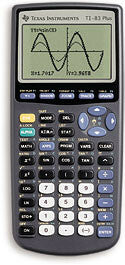# TI-83 Plus Calculator (Used)

• \$50.00
Unit price per
Shipping calculated at checkout.

Operations/Functionality:
• Included Apps - Science Tools, StudyCards™, Probability Simulation, Vernier EasyData®
• TI-GRAPH LINK cables and TI Connect software allow information to be transferred to a computer and printed or stored on disk.
• Apps are available from TI and other leading developers.
• 8-line by 16-character display.
• Real and complex numbers calculated to 14-digit accuracy and displayed with 10 digits plus a 2-digit exponent.
• Graphs 10 rectangular functions, 6 parametric expressions, 6 polar expressions and 3 recursively-defined sequences.
• Up to 10 graphing functions defined, saved, graphed and analyzed at one time.
• Sequence graphing mode shows time series plot, cobweb/ stair-step plot and phase plots.
• User-defined list names. Lists store up to 999 elements.
• 14 interactive zoom features.
• Function evaluation table shows numeric evaluation of functions in table format.
• Interactive analysis of function values, roots, maximums, minimums, integrals and derivatives.
• 7 different graph styles for differentiating the look of each graph drawn.
• Horizontal and vertical split- screen options.
• Matrix operations including inverse, determinant, transpose, augment, reduced row echelon form and elementary row operations. Convert matrices to lists and vice-versa.
• List-based one- and two-variable statistical analysis, including logistic, sinusoidal, median-median, linear, logarithmic, exponential, power, quadratic polynomial, cubic polynomial, and quadratic polynomial regression models.
• 3 statistical plot definitions for scatter plots, xy-line plots, histograms, regular and modified box-and-whisker plots, and normal probability plots.
• Advanced statistics features including 9 hypothesis testing functions, 6 confidence interval functions and one-way analysis of variance.
• 15 probability distribution functions including Normal, Student-t, Chi-square, Binomial and Poisson.
• Business functions including Time-Value-of-Money (TVM), cash flows, and amortization. Full screen interactive editor for solving TVM problems.
• Interactive equation solver editor for solving for different variables in an equation.
• Alphabetical CATALOG of all TI calculator operations in one menu.
• Programming capability with the number of programs limited only by available memory.
• Link with another TI-83, TI-83 Plus or TI-84 Plus. Receive lists L1-L6 from TI-82.